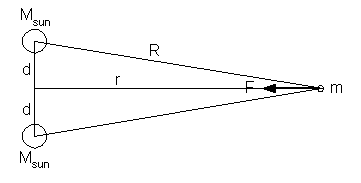# Binary Star System: Kinetic Energy of an approaching Comet

A binary star system has two stars, each with the same mass as our sun, separated by 1.6x10^12 m. A comet is very far away and essentially at rest. Slowly but surely, gravity pulls the comet toward the stars. Suppose the comet travels along a straight line that passes through the midpoint between the two stars. What is the comet's speed at the midpoint?

I'm confused as to how to approach this problem.

I realized quickly that the potential energy of the comet at the midpoint is 0 because at that point the radius equals 0, so I wanted to use kinetic energy and work to figure the problem out, the only problem is that I don't know the distance over which the force is exerted.

Also, I know the force that the stars are exerting on the comet using F_g=G*m_1*m_2/r^2. Well I guess it's more that I know the acceleration due to gravity that the comet experiences because I can cancel out the mass of the comet on both sides, which is necessary because the problem doesn't state the mass of the comet. I could then figure the problem out using the kinematic equation v^2=v_0^2+2aΔx, but I don't know the distance from the star system that the comet starts off at.

Now that I think about it, there must be a maximum radius where the star system starts acting on the comet, and I can use that as my distance... actually if I can determine that I think I will just say that the initial potential energy=final kinetic energy and use that maximum radius as my "height."

Specific Questions:
Can I treat the star system as one source of gravitational force where the radius is the comet's distance from the center of mass of the two stars?
Is there a specific method for solving this kind of problem that I'm not aware of?
Is my last paragraph "Now that I think about it..." on the right track?

Okay so that method might still work, but using the function g=G*m/r^2 when is g close enough to 0 to say that the comet is not being acted on by the gravitational constant of the stars?

To begin, there is no maximum radius. Gravity is an infinite range force. It falls to zero as r approaches infinity.
Second, I definitely think that using distance to the center of mass of the two stars as your radius is the way to go.
Now, the potential at the center is NOT zero, it's infinite. $U \propto \frac{1}{r}$ So if r is zero, U is infinite.
Do you know if your professor wants a number for an answer? As far as I can tell, you need a distance to carry out this calculation. Otherwise, you can solve the equation for any given initial distance. I may be missing something more, might have to think on this one.

gneill
Mentor
You can integrate F.distance from "infinity" to r = 0 if you pay attention to the geometry.
Let d be the distance to the centerline between the stars. The distance to the comet along that centerline is r. The distance from either star to the comet is R.Both stars will exert a gravitational force on the comet, the net force F being aligned along the bisector r. The "vertical" components cancel leaving only the force along r.

Write the expression for the net force F on the comet at distance r. The force vector should lie along the bisector.